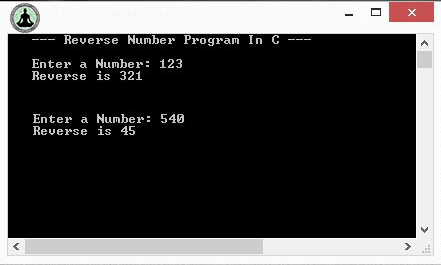# C Program To Reverse a Number

Write a program to reverse a number or digits in C, a very basic and commonly asked question in interviews and exams. Today you will learn the standard method to reverse the number or digits and later we will tell you the error or bug which ever reverse number program have and how to remove that error completely!

Source Code:
```#include <stdio.h>
#include <conio.h>

void main(){
int num , rev=0;
printf("--- Reverse Number Program In C ---\n\n");
printf("Enter a Number: ");
scanf("%d",&num);
while(num>0){
rev = rev*10 + num%10;
num = num / 10;
}
printf("Reverse is %d",rev);
getch();
}

```

Output:ERROR / BUG:
The above program work perfectly but when you enter a number having 0 (zero)s at the end than you will find out what's wrong with this program! ( See above output image carefully )
e.g If you enter 540 than the reversed number will be 45 instead of 045.

Correction:
So the only thing we have to do is count the leading number of zeros and print them before the reversed number. Lets see how to do that!

Correct Source Code:
```#include <stdio.h>
#include <conio.h>

void main(){
int num , temp, rev=0, c=0, i=0;
printf("--- Reverse Number Program In C ---\n\n");
printf("Enter a Number: ");
scanf("%d",&num);
temp = num;
while(num>0){
rev = rev*10 + num%10;
//Check for zeros
if(num%10==0 && i==0) c++;
else i=1;
num = num / 10;
}
printf("Reverse is ");
for(i=0;i<c;i++){
printf("0");
}
printf("%d",rev);
getch();
}
```
Output:Q: write a program to print the following
user input:mirror
required output is:mirorI guess you missed our reply to this question....
visit-> Hereif i give number as 1234 then i want the reverse of a number as3124 . help me@Prashanth you just want to change the location of last digit?we can also use this simple logic for above program
while(n>0)
{

r=n%h;
printf("%d",r);
n=n/h;

}
but i want code if we give the number n=00001123300
output=00332110000 i want out put like this if you have code plzz send me the code to
my email-:neelapuviny9989@gmail.comh=10This is helpful for a situation in which the input has trailing zeroes and you want to reverse them into leading zeroes, but what about when the input has leading zeroes and you want to reverse them to trailing zeroes? Example:
Input: 001234
Output: 432100
This is where I am truly stuck. The only way I can think of doing it is by turning the input number into a string, but then I personally don't know how to do it through that method either. This is a really tricky problem as a beginner.